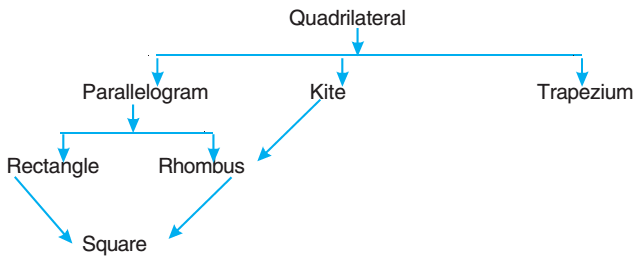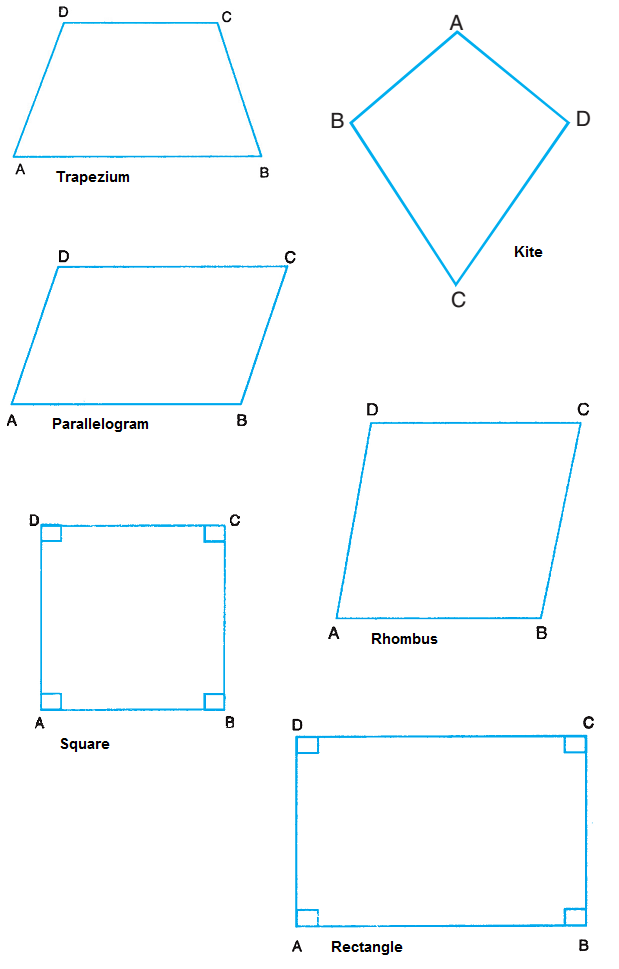Quadrilateral types are parallelogram, kite, trapezium, rectangle, rhombus and square.

A family tree of quadrilaterals is:### Trapezium

A quadrilateral which has only one pair of opposite sides parallel is called a trapezium.

### Kite

A quadrilateral, which has two pairs of equal sides next to each other, is called a kite.

### Parallelogram

A quadrilateral which has both pairs of opposite sides parallel, is called a parallelogram.

• The opposite sides of a parallelogram are equal.
• The opposite angles of a parallelogram are equal.
• The diagonals of a parallelogram bisect each other.

### Rhombus

A rhombus is a parallelogram in which any pair of adjacent sides is equal.

• All sides of a rhombus are equal.
• Opposite angles of a rhombus are equal.
• The diagonals of a rhombus bisect each other at right angles.

### Rectangle

A parallelogram one of whose angles is a right angle is called a rectangle.

• The opposite sides of a rectangle are equal.
• Each angle of a rectangle is a right angle.
• The diagonals of a rectangle are equal.
• The diagonals of a rectangle bisect each other.

### Square

A square is a rectangle, with a pair of adjacent sides equal. In other words, a parallelogram having all sides equal and each angle a right angle is called a square.

• All the sides of a square are equal.
• Each of the angles measures 90°.
• The diagonals of a square are equal.
• The diagonals of a square bisect each other at right angles.